# Computer Class 12 GSEB Solutions Chapter 7 Java Basics

∧Students frequently turn to Computer Class 12 GSEB Solutions and GSEB Computer Textbook Solutions Class 12 Chapter 7 Java Basics for practice and self-assessment.

## GSEB Computer Textbook Solutions Class 12 Chapter 7 Java Basics

Question 1.
What is the size of character data type in Java ?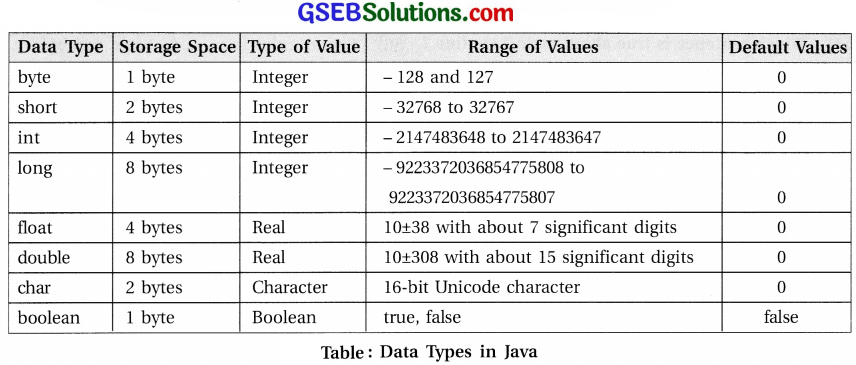Question 2.
Write the number of bytes taken by int and long data types in Java.Question 3.
Explain if and switch statement available in Java.

• The if statement when used in a program enables to take one of two alternative courses of action, depending on whether the value of a given Boolean-valued expression is true or false.
• It is an example of a “branching” or “decision” or “selective” control structure.
• The form if statement is as shown in figure .• When if statement is executed, it first evaluates the Boolean expression.
• If its value is true, it executes statemetl (all statements in a block); otherwise it executes a block of statements written after keyword else.
• <statement> in if statement is usually a block statement.
• It may be any single statement, but it is advisable to use block to make it easy to insert other statements later.
• Refer following code snippet that uses if statement to determine whether an integer is even or odd.
if (x%2 == 0) // assume int x
{ // divisible by 2
System.out.print(x);
System.out.println(" is even");
}
else
{ // not divisible by 2
System.out.println(x + " is odd");
}
• Keyword else and a block after else are optional.
• So, if statement can be without else part as shown in the following form :
if( <boolean-expression>)
{
<statementl>
}
• When an if statement is used in another if statement, it is called nested-if statement.
Example-6 : The following example determines grade based on marks obtained.
if( marks >=70 ) // int marks
}
else
{
if (marks >= 60)
else if (marks >= 50)
else
}
• Now, consider one more example of nested-if statement.
if ( x > 0 )
if (y > 0)
System.out.println("both x and y are greater than zero");
else
System.out.println("x <= 0");
• Here, it seems that the else part is corresponding to “if(x>0)” statement, but actually it is attached to “if(y > 0)”, which is closer.
• Thus it is executing the true part of (x>0).
• If an ‘else’ has to be attached to “if (x>0)”, then enclose the nested if in a block as shown below :
if ( x > 0 )
{
if (y > 0)
System.out.println ("both x and y are greater than zero");
}
else
System.out.println("x <= 0");

Switch Statement :

• A switch statement is used when there are many alternative actions to be taken depending upon the value of a variable or expression.
• Here, the result of the test expression must be of the type taking discrete values.
• The form of switch statement is shown below :
switch ( <expression>)
{
case <constant-1>
statements-1>
break;
case <constant-2>
<statements-2>
break;
// more such cases
case <constant-n>
<statements-n>
break;
default:
<statements-n1>
}
• In the switch statement, the test expression should be of the type byte, char, short or int. It can also be of enum data type.
• When executing switch statement, the value of the test expression is compared with each of the case values in turn from easel onwards.
• If a match is found, the respective case statements (not necessarily a block) are executed.
• If no match is found, the default statement is executed.
• The default is optional, so if there is no match in any of the cases and the default doesn’t exist, the switch statement completes without doing anything.
• Note that break statement used after each case is not mandatory.
• Use of break statement is to break the switch statement, i.e. to jump at the first statement after the end of switch.Question 4.
Discuss about various repetitive structures available in Java.

• Java supports three types of looping constructs: for, while and do…while.
• Figure shows the syntax and structure of all these loops.• In for and while loop, test expression is evaluated first and the statements in the loop are executed if condition is true.
• These loops are entry-controlled or pre-test loop constructs.
• Here, it is possible that statements in a loop are not executed at all.
• When number of iterations are pre-determined, usually for loop is used.
• In this loop, all the three expressions are optional.
• First expression is initializer, second expression is condition and the third expression is an iterator.
• Thus for(;;) is valid; but requires some control statements to break the loop.
• If break statement is not executed, it will result in infinite loop.
• In do…while loop, it evaluates the test expression after executing the statements in a loop.
• It repeats the loop if the test condition is true.
• Thus, do…while loop is exit controlled or post¬test loop construct.
Here, statements of loop are executed at least once.
• Following examples print integer values from 0 to 9 using all the three loop constructs.
• Use of for loop :
for(int i = 0; i < 10; i++)
{
System, out.println(i)
}
• While loop :
int i = 0;
while (i<10)
{
System.out.println(i++); // prints I before applying ++
}
• Use of do…while loop :
int i = 0;
do
{
System.out.println (i++);
} while(i<10);

Use of break and continue statement :

• The break statement is used to transfer the control outside switch or loop structure.
• When break is used in switch structure, it skips all the following statements and control is transferred at the first statement after the end of switch statement.
• In a loop, break statement is used to exit the loop.
• When break is executed in a loop, all the following statements in a body of the loop are skipped and no further iteration takes place.
• The control is transferred at the first statement after the end of loop structure.
• Break jumps outside the nearest loop containing this statement.
• Use of continue statement is used to skip the following statements in a loop and continue with the next iteration.
• Both the statements break and continue are used the same way as in C language.

Nested loops :

• Loops of same or different types can be nested in Java.
• Figure shows the use of nested loops, break and continue statement.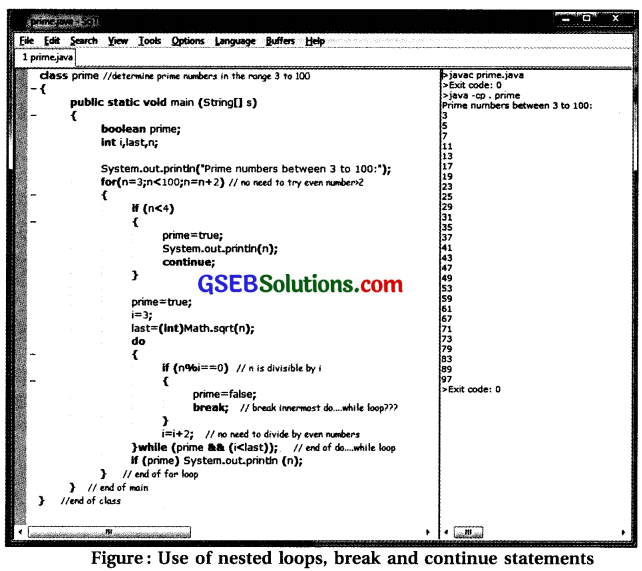• In figure the program prints prime numbers between 3 and 100.
• Additionally, it also shows the use of sqrt function.
• The Java class libraries include a class called Math.
• The Math class defines a whole set of math operations.
• Function sqrt() is one of the static method member of the class Math and is invoked as Math.sqrtO.

Labelled loops and labelled break :

• When there is a use of nested loops, break statement breaks the nearest enclosing loop and transfers the control outside the loop.
• Similarly continue also restarts the enclosing loop.
• If the programmer wants to control which loop to break and which loop to reiterate, labelled loop can be used.
• To use a labelled loop, add the label followed by colon (:) before the loop.
• Then add the name of the label after the keyword break or continue to transfer control elsewhere other than enclosing loop.
• Figure shows a program that uses labelled loops.
• When code in figure is executed, the value of i*x is 350 when i=5 and x-70.
• As the if condition in while loop is evaluated to true, it executes ’break out;’ statement.
• This will terminate the outer for loop labeled as ’out’.
• If only break statement would have used, it would have exited an enclosing while loop and continues with next iteration in for loop.
• Here, it does not execute outer loop for i = 6 onwards.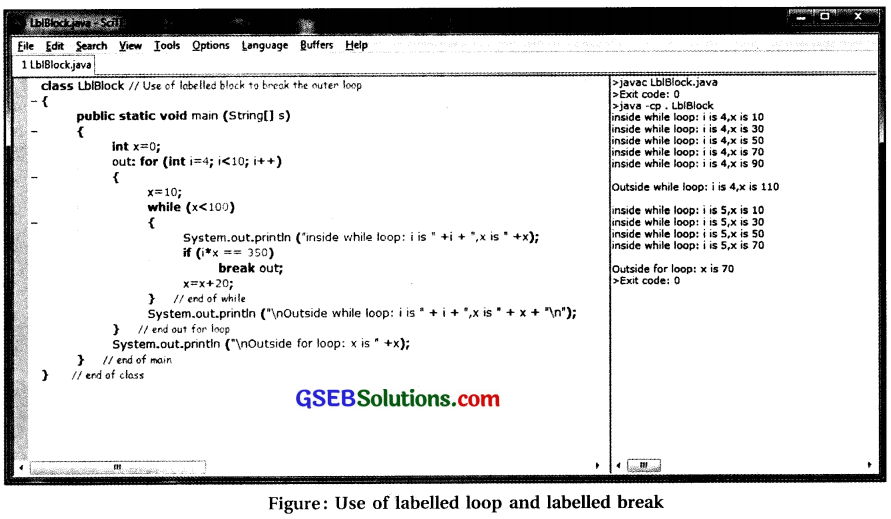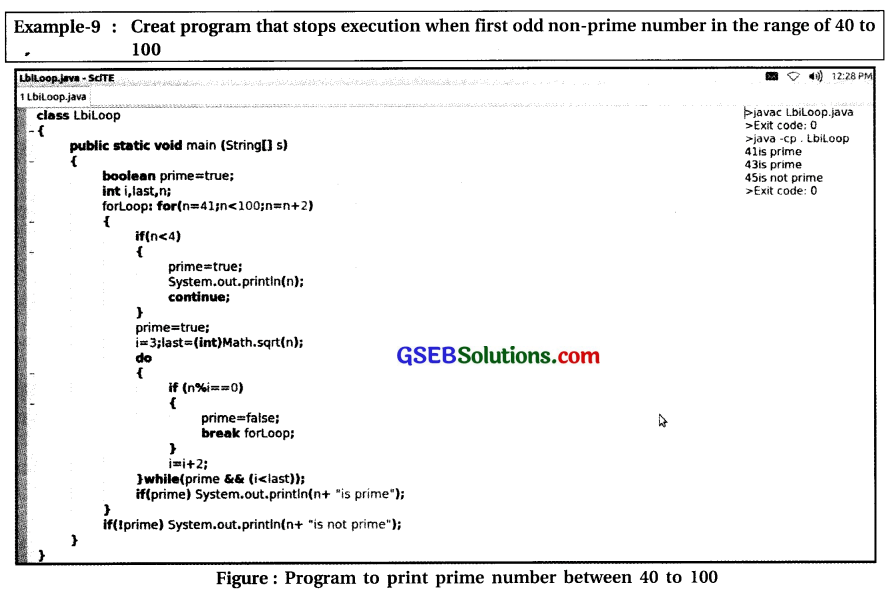Question 5.
How can one use labels for a block or loop in Java ?

• A block statement is a group of statement enclosed between a pair of braces, “{” and “}”.
• The format of a block is :
{
<statements>
}
• Block can be used for various purposes as follows :
• 1. To group a sequence of statements into a unit that is to be treated as a single statement, usually in control structures.
• 2. To group logically related statements.
• 3. To create variables with local scope for statements within a block.
• For example,
{ // This block exchanges values of x and y
int temp; // temporary variable for use in this block only
temp = x; // save a copy of x in temp
x = y; // copy y into x
y = temp; // copy temp into y
}
• When we declare variables inside a block, they are local in that block and such variables will cease to exist after the block.
• We cannot use temp outside the block in which it is declared.
• A variable declared inside a block is completely inaccessible and invisible from outside that block.
• When the variable declaration statement is executed, memory is allocated to hold the value of the variable.
• When the block ends, that memory is released and is made available for reuse.
• The variable is said to be local to the block.
• There is a general concept called the “scope” of a variable.
• The scope of a variable is the part of the program in which that variable is valid.
• The scope of a variable defined inside a block is limited to that block only.
• When the programmer tries to declare a variable with the same name as of the variable in scope, there will be an error.
• Check code listing and the result shown in figure. Here there is a use of labeled block only for better understanding.
• In code listing shown in figure declare variable x in block labelled blk2. Block blk2 is in the scope of main method block. Variable x is declared in the block of main method is also having its scope in blk2. Thus declaring variable x in blk2 conflicts with variables x in the scope of main method block and compiler shows an error.• Figure shows the modified code.
• Here variable x is declared in the scope of entire main method.
• As blkl and blk2 are in the same block, x is available in blkl and blk2 as well.
• Variable y is declared in labeled block blkl, ceases to exist outside that block.
• After execution of blkl, variable y is discarded.
• In blk2, when variable y is declared, it may be allocated any memory location from available memory.
• If one does not initialize value of variable y in blk2, it will return an error when we refer it in blk2.
• This means that variable y of blk2 has nothing to do with variable y of blkl.
• Actually variable y of blkl is not accessible in blk2.Question 6.
Choose the most appropriate option from those given below :

1) How many basic (primitive) data types are supported in Java ?
(A) 2
(B) 4
(C) 8
(D) 16
(C) 8

2) What is the default data type of floating point literal ?
(A) int
(B) long
(C) float
(D) double
(D) double3) Which character set is used for char data type in Java ?
(A) Unicode
(B) ASCII
(C) EBCIDIC
(D) All of these
(A) Unicode

4) Which of the following is compiled error free ?
(A) for(;;){int i=7};
(B) while (1) {int i=7};
(C) while (True) {int i=7};
(D) All of these
(D) All of these

5) Which of the following is not allowed as first character in valid variable name ?
(A) Underscore (_)
(B) Digit
(C) Letter
(D) Dollar ($) Answer: (B) Digit 6) Which of the following is not a basic data type in Java ? (A) char (B) long (C) byte (D) string Answer: (D) string 7) What is the default value of boolean type data ? (A) null (B) true (C) false (D) 0 Answer: (C) false 8) What will be the result of arithmetic expression 7/2 ? (A) 3 (B) 3.5 (C) 1 (D) 0 Answer: (B) 3.5 9) What will be the result of arithmetic expression -7%2 ? (A) -3 (B) -1 (C) 1 (D -3.5 Answer: (B) -110) What will be the result of arithmetic expression -7.5%2 ? (A) -3 (B) -1.5 (C) 1.5 (D) Error Answer: (B) -1.5 ### Computer Class 12 GSEB Notes Chapter 7 Java Basics Introduction to Java • Java is an object-oriented programming language developed by Sun Microsystems, a company best known for its high-end Unix workstations. • Modeled after C++, the java language was designed to be small, simple and portable across platforms and operating systems, both at the source and at the binary level. • Java language was developed at Sun Microsystems in 1991. • Java is small, fast, efficient and easily portable to a wide range of hardware devices. • It is considered as one of the ideal language for distributing executable programs via the World Wide Web and also a general-purpose programming language for developing programs that are easily usable and portable across different platforms. • Java is an object-oriented language and here it differs from C. • Using Java, one can take full advantage of object oriented methodology and its capabilities of creating flexible, modular and reusable code. • It includes a set of class libraries that provide basic data types, system input and output capabilities and other utility functions. • These basic classes are part of the Java Development Kit (JDK). JDK has classes to support networking, common Internet protocols and user interface toolkit functions. • Because these class libraries are written in Java, they are portable across platforms as all Java applications are. • Java is platform-independent at both the source and binary level. • Platform-independence is a program’s capability of being moved easily from one computer system to another. • At the source level, Java’s primitive data types have consistent sizes across all development platforms. • At binary level, platform-independence is possible due to bytecode interpreter. • The designers of Java chose to use a combination of compilation and interpretation. • The scenario of platform independence is shown in figure.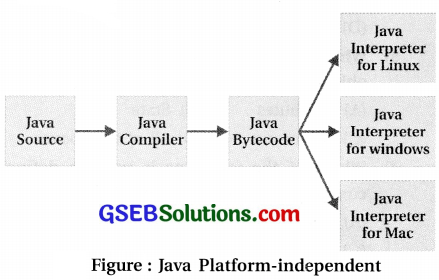• Programs written in Java are compiled into .machine language for a computer that doesn’t really exist. • This so-called “virtual” computer is known as Java Virtual Machine (JVM). • The machine language for the Java Virtual Machine is called Java Bytecode. • Different Java bytecode interpreter is needed for each type of computer. • Java binary files are actually in a form called bytecodes that is not specific to any one processor or any operating system. • The only disadvantage of using bytecodes is its slow execution speed. • There are tools available tools to convert Java Bytecodes into native code. • Native code is faster to execute, but then it does not remain machine independent.Creating Simple Java Application • A Java program is composed of classes. • It should have at least one class and it must have main method in it. • C programmers can think of a class as a sort of creating a new composite data type by using struct and typedef. • Classes, however, can provide much more than just a collection of data. • Note that typedef is not available in Java. • Let us now create a Java application with a name ‘CallCost’ that computes call charges and updates balance. To create and execute ‘CallCost’ application, the following steps have to be performed. (1) Create Java source file using any plain ASCII text editor. • Choose any text editor and type the program given in code listing. • Save the source file with name ‘CallCost. java’. Conventionally, Java source files are given the same name as the class defined, with an extension of java. • Note that the class name and filename are case sensitive. So, if class name is CallCost, filename should be CallCost.java (Applicable to SciTE editors). (2) Compile the source file using the Java compiler. • To compile the Java program, type javac followed by the name your source file: javac CallCost.java. • If the compiler show any errors, go back and make sure that the program is typed exacdy as it appears in code listing. • When the program gets compiled without errors, compiler creates a file with extension .class in the same directory as your source file. • Checks that the compiler has created file ‘CallCost.class’. • This is the Java bytecode file that will be executed. (3) Run the application using Java interpreter. • In JDK, the Java interpreter is called by simply using java. • Type java CallCost. • Interpreter java uses the bytecode CallCost.class and executes it. / ** * This class implements a simple program that * will compute the cost of phone call and update balance */ public class CallCost { public static void main (String [] args) { /*declare variables */ double balance; // balance amount in rupees double rate; // call rate; rupees per second double duration; // call duration in seconds double cost; // cost of last call /*computations. */ balance = 170; rate = 1.02; duration = 37; cost = duration * rate; // compute the cost balance = balance - cost; // update balance amount /*display results */ System.out.print("Call Duration: "); System.out.print (duration); System.out.println("Seconds"); System.out.println("Balance:" + balance + "Rupees "); } // end of main() } // end of class CallCost Code Listing : Sample Java Program • The process of compiling and executing a Java application is shown in figure.• Java source program file should have extension .java. • Its name should be same as that of class when class is public. • Note that the name is case sensitive. • The compiler ‘javac’ compiles source file and creates Bytecode file. • The name of bytecode file is same as the class name containing main method and it has an extension ‘.class’. • An interpreter ‘java’ interprets bytecode and excutes it. • Figure shows how to compile and execute the program in Linux environment using a terminal.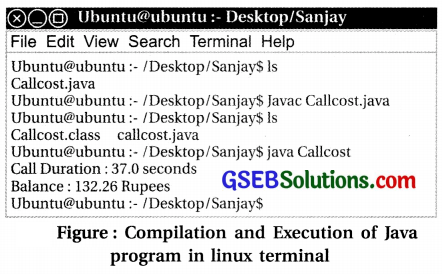• Notice two main parts in code listing. 1. The program is enclosed in a class definition; here, a class called ‘CallCost’. 2. The body of the program is contained in a routine called main(). In Java applications, main() is the first routine that is run when the program is executed. • Explanation of code Listing: • The text written after / and enclosed within /* and */ are comments. • Comments are not compiled or interpreted. • Variables are declared using data type followed by variable name. • Computation part contains expressions including assignment statements. 1. Here, several subroutine (also called function or method in Java) call statements are used to display information to the user of the program. 2. Methods used to display results : System.out.print and System.out.println. Both these methods take a value to be displayed as an argument. 3. Method System.out.println adds a linefeed after the end of the information that it displays while System.out.print does not. 4. Call duration is displayed using three calls. 5. First call displays label ‘Call Duration:’, second call displays the value of the variable ‘duration’, third call displays label ‘Seconds’ and then bring the cursor in the next line. Note that string literal is enclosed in double quotes. 6. Balance is displayed using single call. Notice the use of +operator in Java in the parameter passed. It first evaluates the expression given as parameter and then display. • When the program is run, the Java interpreter calls the main() method and the statements that it contains are executed. • These statements tell the computer exactly what to do when the program is executed. • The main() routine can call other subroutines that are defined in the same class or even in other classes, but it is the main() routine that determines how and in what the other subroutines are used. • The word “public” in the first line of mainO means that this routine can be called from outside the program. • This is essential because the mainO routine is called by the Java interpreter, which is external to the program.Using Scite • SciTE editor is used to create Java application here. • The application here computes simple interest and displays the results. • Figure shows the code listing and its output. 1. Start SciTE application. Select File → New. 2. Type the Java program as per code listing given in figure and save the source program in a file with the name Interest.java. 3. To save the file, select File → Save command. 4. Compiler source program using Tools → Compile command. 5. If the program is compiled without any error, execute it using Tools → Go command.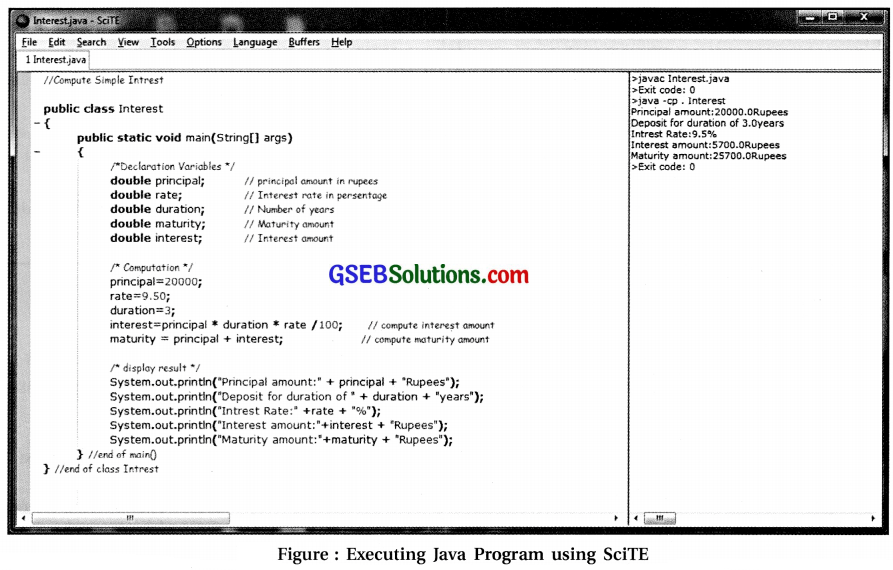Structure of a Java Program • Programming languages differ from ordinary human languages in being completely unambiguous and very strict about what is and is not allowed in a program. • The rules that determine what is allowed are called the syntax of the language. • Syntax rules specify the basic vocabulary of the language and how programs can be constructed using things like variables, expressions, statements, branches, loops and methods. • A syntactically correct program is one that can be ‘ successfully compiled or interpreted. • A structure of Java program is shown in figure . • Here, text in angle bracket < and > is used as a placeholder that describes something actual we need to type while writing actual program. • The definition of the method (function) in Java consists of function header and the sequence of statements enclosed between braces { and }. • As Java is an object-oriented language. Everything is defined as part of class. • Thus a method can’t exist by itself. It has to be a part of a class. public class <class-name> { <optional-variable-declaration-and-methods> public static void main(String[] args) { <statements> } <optional-variable-declarations-and-methods> } Figure : Structure of a Java Program • <class-name> in the first line is the name of the class having main method in it. • If the name of the class is CallCost, then the program should conventionally be saved in a Java source file with a name CallCost.java. • When this file is compiled, another file named CallCost.class is generated. • This class file is given a name using class name. • Class file, CallCost.class, contains the translation of the program into Java bytecode, which can be executed by a Java interpreter. • Variable and method declaration after and before main() method is optional. • Each program must have one class that contains public method main(). • Java is a free-format language. The layout (such as the use of blank lines and indentation) of the program in code listing is not a part of the syntax or semantics of the language. • The computer doesn’t care about the program layout. • The entire program can be written together on single line as far as it is concerned but layout is important to make it more readable. • A program can contain other methods besides main(), as well as other variables. Data Types • Data type determines the required memory size, type of values, range of values and type of operations that can be performed. • Java supports eight primitive data types that handle common types for integers, floating-point numbers, characters and Boolean values (true or false). • The primitive data types are named byte, short, int, long, float, double, char, Boolean. • The first four typed hold integers (whole numbers such as 17, -38477, and0), next two hold real numbers (such as 5.8, -129.35), char holds a single character from the Unicode character set and Boolean holds one of the two logical values true or false. • These data types are called primitive as they are built into the system. • Note that these data types are machine- independent, which means that we can rely on their sizes and characteristics to be consistent across all Java programs on all machines. • Table 7.1 lists the details of data types.• Integer numbers with b bits precision store signed values in the range of (-2b – 1 -1, 2b – 1). • When they are preceded with keyword unsigned, the values are in the range of (0, 2b – 1). • Real numbers in Java are complaint with IEEE 754 (an international standard for defining floating¬point numbers and arithmetic). • Java uses the Unicode character set. • The char type has 16 bits of precision and is unsigned. • This allows thousands of characters from many different languages and different alphabets to be used in Java. • Data type Boolean is not a number, nor can it be treated as one.Variable • If anything is to be remembered by the computer, there will be a requirement of variables during program execution. It needs to be stored in the memory of the computer. • Programs manipulate the data that are stored in memory. • In machine language, data can only be referred to by giving the numerical address of the location in memory where it is stored. • In a high-level language such as Java, names are used instead of numeric address of memory location to refer to data. • The programmer has to remember only the name. • A name is used to refer to the data stored in memory and it is called a variable. • A variable can take different data values at different times during the execution of the program, but it always refers to the same memory location. • A variable can be used in a java program only if it has been declared. • One or more variables can be declared in Java using declaration statement with following syntax. <type-name> { variable-names}; • The conventions used here in the syntax are as follows : 1. Angle brackets < > denote the item to be specified by user. 2. Curly brackets { } denote the list of items separated by commas. • Here {variable names} denote the list of variable names. • When the list contains more than one item, items should be separated by commas. • <type-name> is to be replaced with the keyword denoting the data type of the variables. • It is used to determine the size of variable, the values it can hold and the operations that can be performed on it. • When the computer executes a variable declaration statement, it sets aside memory for the variable and associates the variable’s name with that memory. • Some examples of variables are as mentioned : 1. int marks; 2. double amount, interest; 3. float rate; 4. char grade; 5. boolean isPass; • To define variable name, certain rules have to be followed : • It must begin with an alphabet, underscore (_) or dollar sign ($). After first character, it may contain digits, alphabets, $and underscore. 1. Some legal names are : birth_date, result, CallCost, top5students, date, amount$.
2. Some illegal names are 4me, %discount, birth date.
• No spaces are allowed in variables. Thus birth date is invalid variable name.
• It cannot be a reserved word. Reserved words have special use in Java and cannot be used by the programmer for other purposes. Examples of some reserved words are class, public, static, if, else and while.
• Guidelines for naming variables :
• Choose meaningful names. Java allows variable name of any length.
• When a name includes several words, such as ‘balance amount’, follow one of the conventions mentioned below:
1. Capitalize the first alphabet of each word, except for the first word. This is sometimes referred to as camel case, since the upper case letters in the middle of a name are supposed to look like the hump on a camel’s back. Example: balanceAmount, birthDate.
2. Separate words with underscore. Examples: balance_amount, birthdate.
• Note that Java is case-sensitive. So, upper case and lower case letters are considered to be different. Thus, variable names balance and Balance are different.
• It is customary for names of classes to begin with upper case letters, while names of variables and of methods begin with lower case letters.
• Good programming style for declaring variables :
• Declare only one variable in a declaration statement.
• Include a comment with each variable declaration to explain its purpose in the program.
• Declare important variables at the beginning of the function. Declare variables which are not important to the overall logic of the function at the point where they are first used.
• In Java, there are three kinds of variables: instance variables, class variables and local variables.
• Function parameters and variables declared in the function are considered as local variables.
• Each variable can be given an initial value while declaring.
For example :
– int marksObtained, totalMarks=100, counter=0;
– boolean isPass = true;
• In general, syntax of declaring variables is as follows : <type name>{variable [=<value>,]};
• Here items in square bracket [ ] denotes optional item.
• Note that local variables are not initialized with default values. It is programmer’s responsibility to assign value to such variables before their first use.
• Figure shows the use of variable in java program.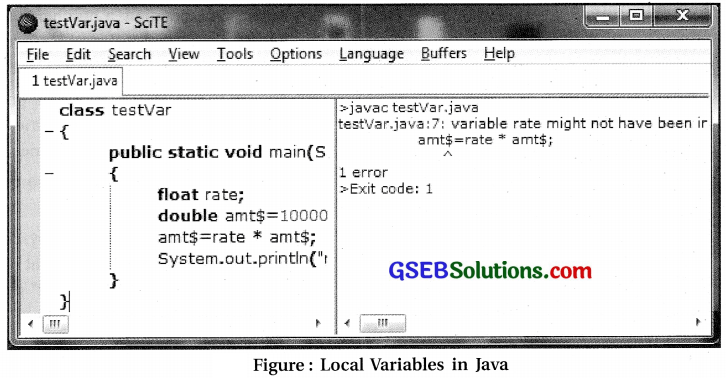• Observe that the local variable ‘rate’ is not , assigned any value before its use in statement ’amt$=rate * amt$’.
• When we try to compile the program the compiler will give an error as can be seen in figure.

Literals

• A name used for a constant value is known as literal.
• There are some different kinds of literals in java for number, character, string and Boolean values.

Numeric Literals :
Numeric literals are used to represent integer or real numbers for example, 157 and 17.42 are literals.

Integer Literals :

• Integer Literals are literals that are whole numbers.
• Java allows decimal (base-10), octal (base-8) and hexadecimal (base-16) and Unicode integer literals.
• Ordinary integers such as 4, 157, 17777 and -32 are decimal integer literals of type byte, short or int depending on their size.
• A decimal integer larger than int is automatically of type long.
• The user can force a smaller number to be long by appending an L or l (upper or lower case letter l) as a suffix to that number (for example, 4L is a long integer having value 4).
• Negative integers are preceded by a minus (-) sign, for example, -45.
• Octal numbers use only the digits 0 through 7. In Java, a numeric literal with a leading 0 (zero) is interpreted as an octal number. For example, literal 045 represents octal integer whose decimal number value is 37.
• Hexadecimal numbers use 16 digits, the usual digits 0 to 9 and the letters A, B, C, D, E and F. Upper case and lower case letters can be used interchangeably in this context.
• The letters A to F represent the numbers 10 to 15 respectively.
• In Java, a hexadecimal literal begins with Ox or OX. Examples of hexadecimal literals are 0x45 or 0xFF7A.
• Hexadecimal numbers are also used in character literals to represent arbitrary Unicode characters.
• A Unicode literal consists of \u followed by four hexadecimal digits. For example, the character literal ‘\u00E9’ represents the Unicode character that is an “e” with an acute accent.
• Java 7 also supports binary numbers, using the digits 0 and 1 and the prefix Ob (or OB). For example OblOllO or OblOlOl 1001011.

Real number literals :

• Real number literals are called floating point literals. These numbers can be represented using two types of notations: standard and scientific.
• In standard notations, the integer part and the fractional part are separated with decimal point (.), for example 12.37.
• In scientific notation, a number is followed by letter e (or E) and a signed integer exponent, for example 1.3el2 and 12.3737e-108.
• The “e122” and “e-108” represent powers of 10. Hence 1.3el2 means 1.3 times 1012 and 12.3737e-108 means 12.3737 times 10“108.
• Scientific format can be used to express very large and very small numbers.
• In Java, floating point literal by default is of the type double. To make a literal of type float, we have to append an “F” or “f as a suffix to the number. For example, “1.2F” specifies literal 1.2 to be considered as a value of type float.• Observe that in figure there is a compilation error as the programmer has assigned 10.2 to variable of float type.
• To make the program work, this statement has been written as “float rate = 10.2f;”.
• The suffix F can be written in either lower or upper case.

Boolean literals :

• For the type Boolean, there are precisely two literals: true and false.
• These literals are to be types without quotes.
• They represent values, not variables.
• Boolean values occur most often as the values of conditional expressions.
• In C, 0 is treated as false and non-zero value is treated as true,
• in Java, literals true and false are not associated with any numeric value.

Character literals :

• Character literals are expressed by a single character surrounded by single quotes: ‘a’, ‘#’3’ and so on.
• Characters are stored as 16-bit Unicode characters.
• Certain special characters have special literals that use a back slash (\) as an “escape character”. Table
7.2 lists the special codes that can represent nonprintable characters, as well as characters from the Unicode character set.
 Escape Code Meaning \n New line \t Tab \b Backspace \r Carriage return \f Form feed (New page) \\ Back slash character \’ Single quote character \” Double quote character \ddd Character represented by three octal digits (d: 0 to 7) \xdd Character represented by two hexadecimal digits ( d: 0 to 9, a to f) \udddd Character represented by Unicode number dddd (d: hexadecimal digit)

String literals :

• In Java all the other data types represent objects rather than “primitive” data.
• A String is a sequence of characters.
• In code listing we have used a string literal: “Balance:”, String literal is a sequence of characters enclosed in double quotes.
• Within a string, special characters can be represented using the back slash notation as given in Table.
• For example, to represent the string value: Many “Congratulations!”, the following string literal has to be typed :
“Many, \”Congratulations!\””.
• Similarly in string “This string bought to you by Java\u2122”, the Unicode sequence \u2122 produces a trademark symbol (™).

• They are entirely ignored by the computer.
• Comments are important to make the program easy to understand for everyone.
• Java supports following types of comments :
1. Single-line comment : It begins with double slashes (//) and extends till the end of line. The computer ignores the // and everything that follows it on the same line.
2. Multi-line comment : It begins with /* and ends with */. This type of comment is usually used to have more than one line as comments. Any text between two delimiters / * and */ is considered as a comment. Comments cannot be nested; that is, we cannot have a comment inside a comment.
3. Documentation comment : These type of comments begin with /** and end with */. They are used for creating API documentation from the code. These are special comments that are used for the javadoc system.
• Other than comments, everything else in the program is required to follow the rules of Java syntax.

Expressions

• Expressions are an essential part of programming.
• The basic building blocks of expressions are literals (such as 674, 3.14, true and ‘X’), variables and function calls.
• Recall that a function is a subroutine that returns a value.
• Simple expression can be a literal, a variable, a function call. More complex expressions can be built up using operators to combine simpler expressions.
• Operators include arithmetic operators (+, -, *, %), comparison operators (<, >, =, …), logical operators (and, or, not…).
• When several operators appear in an expression, there is a question of precedence, which determines how the operators are grouped for evaluation.
• For example, in the expression “A + B * C”, B*C is computed first and then the result is added to A.
• Multiplication (*) has a higher precedence than addition (+). If the default precedence is not what is required, then parentheses are used to explicitly specify the grouping.
• For example, in expression “(A + B) * C”, it adds A and B first then multiplies the result by C.Operators

• Operators are special symbols used to build expression.
• Java supports many types of different operators. Below given are some of them :
1. Arithmetic Operators
2. Comparison Operators
3. Logical Operators
4. Conditional Operators
5. Assignment Operators

Arithmetic Operators :

• In Java, basic arithmetic operators are addition (+), subtraction (-), multiplication (*), division (/) and modulus (%).
• All these operators are binary, they take two operands.
• Operators + and – can be used as unary (taking only one operand) also.
• All operators can be applied on any type of numeric data: byte, short, int, long, float, or double.
• They can also be used with values of type char, which are treated in this context.
• With char type of data, its Unicode code number is considered as its value when it is used with an arithmetic operator.
• Arithmetic operators are described in Table 7.3.
 Operator Meaning Example Result + Addition 2 + 8 10 – Subtraction 2 – 8 -6 * Multiplication 2 * 8 16 / Division 8 / 2 4 % Modulus (Gives remainder after division where quotient is an integer value) 8 % 3 25.8 % 7 2 4.8

Table : Arithmetic Operators :

• Data type of the result after binary arithmetic operation :
• When both operands are of same data type, the data type of the result is same as the type of operands.
1. If both operands are integers, result is an integer. For example, result of 9/2 is 4 and not 4.5. Integer division results into integer value and remainder is discarded.
2. if both operands are float, result is float. Example: 9f/2f results in 4.5.
• When both operands are of different data typeS.
1. First of all, lower range data type is implicitly converted to higher data type to have the same types of operands. This type of conversion is also known as promotion.
2. Now, the result of an expression will be same higher range operand.
3. Example: 4 + 3.5 results in 7.5, 9/2.0 results in 4.5, 9f/2 results in 4.5.

Points to remember :

1. With modulus operator %, if first operand is negative, the result is negative.
2. In Java, % operator can be used with floating point data types also. The result is the remainder after integer quotient. Thus for 25.8 % 7, integer quotient is 3 and remainder is 25.8 (3*7) = 4.8.
3. Operator + can also be used to concatenate a string.
• When operator + is applied with one of the operand of type String, other operand is automatically converted into type String.
• This is also an example of implicit type conversion.
• This type of conversion is seen in code listing in an expression ‘ “Balance: “+ balance’ while printing – balance amount.
• It is also used in code listing shown in figure.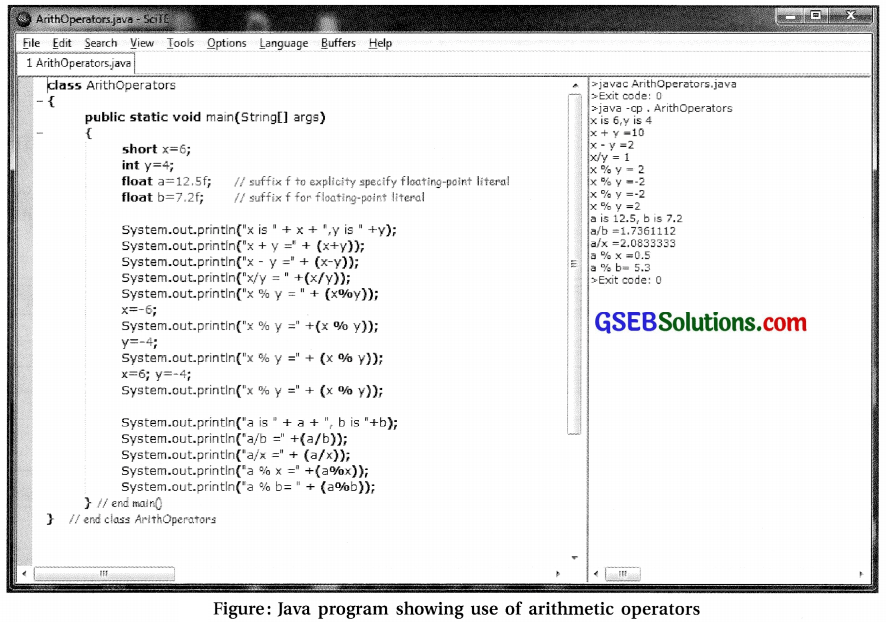Explanation of program shown in figure:

• Initially define four variables in main() method: x and y, which are integers (type short and int); a and b, which are floating point real numbers (type float).
• One has to remember that default type for floating-point literals (such as 12.5) is double. So f is suffixed with literals 12.5 and 7 to treat them as float type.
• The System.out.println()method merely prints a message to the standard output device. This method takes a single argument, a string, but we can use + to concatenate values into a string. As mentioned before, Java converts numbers into string and then concatenates the two.
• The result of x%y is 2 when x-6 and y=-4 because first operand is positive.
• The result of a%x is 0.5 where a=12.5 and x is 6. Here, integer quotient is 2 and the remainder is 0.5. Similarly in case of a%b where a=12.5 and b=7.2; the integer quotient is 1 and the remainder is 5.3.
• See the mixed type of operands in the call: System.out.println (“a / x = ” + (a / x));. Here variable a is float and x is int, the result after division is float. While evaluating an expression (“x+y = ” +x + y) and analyze the results shown in figure.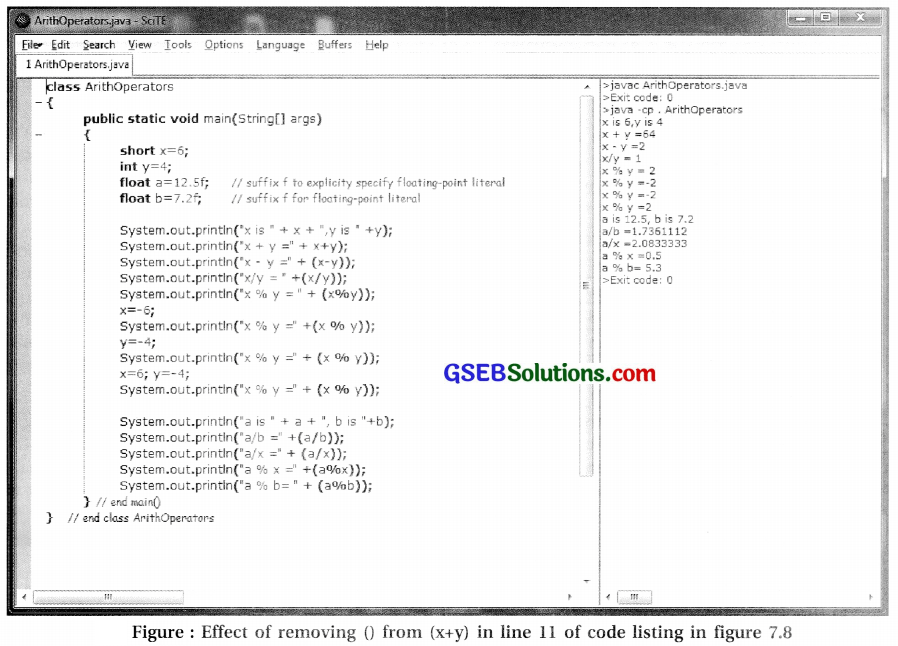Increment and Decrement operators :

• Unary operators ++ and – are called the increment operator and the decrement operator respectively.
• Operator ++ adds 1 to a variable and – subtracts i from the variable.
• These operators can be used on variables belonging to any of the integer types and also on variables
of type char.
• If x is an integer variable, we can use x++, ++x, x–, –x as expressions, or as parts of larger expressions.
• When ++ or – operator is used after variable name, it is known as post-increment or post-dec
operator.
• The old value of variable is used while evaluating the expression and thereafter value of variable is incremented or decremented.
• For example, let the value of variable x be 3 before executing statement y = 4 + x++;. Here the old value of x is used while evaluating expression on right-hand side and thereafter x is incremented. As a result, value of x will be 4 and y will be 7.
• When ++ or – operator is used before variable name, it is known as pre-increment or pre-decrement
operator.
• Here the value of variable is incremented or decremented first and then this new value is used in expression.
• For example, in statement y-4 + ++x;, if old value of x is 3, then value of x is incremented first and then this new value is used in evaluating a right-hand side expression. So value of x will be 4 and that of y will be 8.
• When this operator is used in a standalone , statement, use of pre or post does not make any difference. For example, statements x++; and ++x; are standalone statements.

Comparison operators :

• Comparison operators are also known as relational operators.
• The comparison operators in Java are :
:==, !=, <, >, <=, and >=.
• The meanings of these operators are :
 A==B Is A “equal to” B ? A !=B Is A “not equal to” B ? A < B Is A “less than” B ? A > B Is A “greater than” B ? A <= B Is A “less than or equal to” B ? A >= B Is A “greater than or equal to” B ?
• These operators can be used to compare values of any of the numeric types as well as of char type. For characters, their Unicode numeric values are used in comparison.
• After applying comparison operator, the result of expression is Boolean; either true or false. So, such expressions are also called Boolean-valued expression.
• Boolean-valued expressions can also be assigned to boolean variables, just as assigning numeric values to numeric variables.
• Operators == and != can also be used to compare Boolean values. For example :
• Boolean bothPositive; bothPositive
= ((x>0) == (y>0));
• Usually, relational operators are used in if statements and loops.Logical operators :

• Logical operators are also Boolean operators, as they operate on Boolean operands.
• In Java, logical operations AND, OR, XOR and NOT are performed using operators &&, ||, ∧ and ! respectively.
• Boolean operator && is used to combine values for logical AND operation.
• The result of expression A && B is true only if both the operands A and B are true. For example, (x == 0) && (y == 0) is true if and only if both x is equal to 0 and y is equal to 0.
• Boolean operator for logical OR is || (two vertical line characters). Expression A || B is true if either A is true or B is true, or if both are tme. The result is false only if both A and B are false. For example, (x == 0) && (y == 0) is false only if both x and y are not equal to 0.
• Boolean operator for logical NOT is denoted by ! and it is a unary operator. It results in complemented result. If the operand is true, result is false and vice versa.
• In addition, there is operator A to perform logical XOR (exclusive OR). It returns true only if its operands are different (one true and one false) and false otherwise. Thus result is false when both operands have same Boolean value. For example, (x == 0) ∧ (y == 0) is true if only one of the x or y is zero.

Short circuiting :

When using the conditional AND and OR operators (&& and ||), Java does not evaluate the second operand unless it is necessary to resolve the result.

If the first operand is false in case of &&, there is no need to evaluate second operand.

Similarly, if first operand is true in ||, there is no need to evaluate second operand.

For example, consider expression (x !=0) && (y/x > 1). If the value of x is zero, first sub-expression (x !=0) results in false. As the logical operator && results in false when any one of the operands is false, there is no need to evaluate second sub-expression(y/x > 1). Here, the evaluation has been short-circuited and the division by zero is avoided. Without the short-circuiting, there would have been a ‘division by zero’ error at tuntime.

Conditional Operator :

• The conditional operator in Java is a ternary operator using three operands. It uses two symbols? and : in the expression to delimit three operands. It takes the following form :
<Boolean-expression> ? <expressionl> : <expression2>
• Here, the first operand is a Boolean expression and is evaluated first. If its true, value of the entire expression is the value of second operand expressionl; otherwise it evaluates to third operand expression2.
• For example, consider statement:
next = (N % 2 == 0) ? (N/2) : (3*N+1);
• Suppose value of N is 8. The value of first operand (N % 2 == 0) is true, so value of right- hand side expression is the value of expression (N/2), i.e. 4.
• If N is odd, first expression results in false and it will assign the value (3*N+1) to next. Note that the parentheses in this example are not required, but they do not make the expression easier to read.

Assignment

• In Java, an expression containing assignment ( = ) operator is generally referred to as assignment statement.
• Once a variable has been declared, a value can be assigned to that variable by using the assignment operator ‘=’.
• In Java, one of the ways to get data into a variable is with an assignment statement.
• An assignment statement takes the form :
< variable > = < expression >;
• Where < expression > represents anything that refers to or computes a data value.
• When an assignment statement is executed, it first evaluates the expression on right side of = sign and then put the resulting data value into the variable on left side of = sign.
• For example, consider the simple assignment statement: rate = 10.02f;
• Here the variable in this assignment statement is ‘rate’, and the expression is the number ‘10.02f. Execution of this statement replaces the value of float variable ‘rate’ by 10.02.
• Now, consider another assignment statement: balance = balance – cost;
• Here expression ‘balance-cost’ is evaluated first using the existing values of variables ‘balance’ and ‘cost’ in memory. There after the resulting value is placed invariable ‘balance’ replacing its older value.
• When a variable is used in an expression on right- hand side, it refers to the value stored in the variable.
• When a variable is used on the left-hand side of an assignment statement, it refers to memory location that is named by the variable to place the value.
• So, variable on left-hand side of expression is called lvalue, referring to location in memory.
• In general, the type of the expression on the right-hand side of an assignment statement should be the same as the type of the variable on the left-hand side.
• However, if they are not matching, the value of expression is automatically converted to match the type of the variable.
• If the data type of expression is larger than the variable on left-hand side, it may result in an error due to precision problem. For example, there may not be automatic conversion from int to short or double to float.

Shorthand assignment operators :

• Java also support shorthand version of assignment.
• It saves the typing time,
• It takes the form <variable> <operator> = <expression>.
• Its effect is same as <variable> = <variable> <operator> <expression>.
• Here the operator should be a binary operator using two operands.
• Some examples of shorthand assignment operators are; a += b and q &&= p.
• Here a += b is same as a = a + b, while expression q &&=p is same as expression q = q && p

Type cast

• In some cases, the programmer may want to force a conversion that wouldn’t be done automatically.
• For this a type cast can be used.
• A type cast is indicated by putting a type name in parentheses before the value we want to convert.
• Thus it takes a form : (cdata-type>) cexpression>
• For example
int a; short b;
a = 17; b = (short)a;
• Here variable a is explicitly converted using type cast to a value of type short.

Precedence and associativity of Java operators :

• Java has well defined rules for specifying the order in which the operators in an expression are evaluated when the expression has several operators.
• The operators are evaluated as per their priority (or precedence).

Precedence Order :

• When two operators are having different priority, then an operator with the higher precedence is operated first.
• For example, in expression a + b * c, multiplication is having higher precedence than addition, so b*c is evaluated first and then the result is added to a.
• Thus it is as good as writing a + (b*c).
• Precedence mles can be overridden by explicit parentheses. So, instead of creating confusion, use of parenthesis should be done freely.

Associativity :

• When two operators with the same precedence appear in the expression, the expression is evaluated according to its associativity.
• Associativity determines the direction (left-to- right or right-to-left) in which operations are performed.
• In most of the cases, associativity is from left to right.
• For unary operations and assignment, it is from right-to-left.
• Table 7.4 gives the list of operators discussed in this chapter, listed in order from highest precedence (evaluated first) to lowest precedence (evaluated last);
 Operations Operators Associativity Unary operations ++, –, !, unary – and +, type cast Right-to-left Multiplication, division, modulus *, ?, % Left-to-right Addition and subtraction + , – Left-to-right Relational operators <, >, <=, >= Left-to-right Relational operators (Equality and inequality) ==, != Left-to-right Logical AND && Left-to-right Logical OR || Left-to-right Conditional operator ? ; Right-to-left Assignment operators =, +=, -=, *=, /=, %= Right-to-left

• For example x = y = z = 7is treated as x = (y = (z = 7)), leaving all three variables with the value 7.
• This is due to right-to-left associativity of assignment (=) operator.
• An assignment is an operator, so assignment statement evaluates to the value on the right hand side expression.
• Thus, in x=y=z=7, it evaluates z=7 first.
• This assigns value 7 to variable z and value of expression z=7 is also 7, which is making the expression as x=y=7.
• On the other hand, 72 / 2 / 3 is treated as (72 / 2 ) / 3 since the / operator has left-to- right associativity.
• It should be noted that there is no explicit operator precedence table in the Java Language Specification and different tables on the Web and in textbooks disagree in some minor ways.Control Structures

• In general, the statements are executed sequentially, one by one.
•  Sometimes, program logic needs to change the flow of this sequence.
• This statements that enable to control the flow of execution are considered as control structures.
• There are two types of control structures: loops and branches.
• Loops are used to repeat a sequence of statements over and over until some condition occurs.
• Branches are used to choose among two or more possible courses of action, so also called selective structure.
• In Java, control structures that are used to determine the normal flow of control in a program are: if statement, switch statement, while loop, do..while loop and for loop.
• Each of these structures is considered to be a single statement, may be a block statement.figure